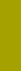﻿ Grain densitySand Texture Sedimentology | Variables

Grain density

Variable 3: Logarithmic Grain Density, LRS

Density is related to specific gravity:

the dimension of density is g/cm3, for specific gravity it is unity

LRS = +log2Rs

LRS is a 3-character abbreviation of log2Rs, where R stands for the Greek letter RHO (ρ), and the subscript s for ‘solid’. The symbol Rs was introduced in classical works of A. T. COREY (1949), E. F. SCHULZ, R. H. WILDE and M. L. ALBERTSON (1954), and has consistently been used in the papers of St. Anthony Falls Labs, such as B. C. COLBY + R. P. CHRISTENSEN (1957), Colorado State Univ. at Forth Collins, Daryl B. Simons, etc..

Our SedVar™ software can efficiently determine a density distribution of equally sized heterogeneous materials, such as a narrow sieved heavy mineral concentrate, without tedious separations as in heavy liquids with various density. Combined density distributions of various sieve fractions provide valuable information about the size-density distribution relationship (available in a three-dimensional, 3D, plot).

Accuracy of the measurement of a density distribution depends on the following factors:

1 Relative equivalence of sieve intervals: if expressed in millimeters, the intervals must form a ge­ometric series, if expressed in PHI notation, they must form an arithmetic series.

2 Narrowness of sieve intervals: sieve intervals smaller than 0.25 PHI are recommended (the millimeter size ratio should be smaller than 1.189 or greater than 0.841);

3 Sieve quality: galvanically deposited (not woven) screens with circular holes are best;

4 Sieving quality: wet sieving increases screening performance (screening with denaturized alcohol permits faster work than with water due to more rapid drying).

5 Limited shape variation of particles coarser than 0.2mm (or of particles with settling rate faster than about 2 cm/sec, = PSI < -1); the settling rate of particles finer than 0.2 mm is almost not af­fected by current shape variation (SF between 1.18 and 0.2).

Factors 3 and 4 improve sieving separation capability which decreases toward finer size; because the best sieving can optimally approach a separation capability of about 3 microns, the almost maximum permissible sieve interval of 0.2 PHI can be extended down toward fine size up to the interval 4.4 to 4.2 PHI (=0.047 to 0.041 mm) which only corresponds to the interval width of 6 microns. Factors 3, 4 and 5 combined result into an optimum size range between 0.08mm to 0.3mm, which allows a sieve size interval of 0.1 PHI or smaller.

If, with coarser particles, the shape variation is so great that it significantly affects the settling rate, the following method helps determine the shape factor:

1     A narrow sieved fraction is separated into narrow density intervals in heavy liquids;

2     Each sieve + density fraction is analyzed by the Macrogranometer™.

3     The resulting PSI distributions of samples with homogeneous size and density are converted into LSF distributions (see the next dispersity variable).

4     The mean SF-values calculated by the LSF program segment can be entered as a “varying constant” for each density interval in order to calculate the density distribution (shape, in some degree, is dependent on a particle’s mineral composition [I. e. density] and size; mean SF-values of each distribution will probably correlate well with LRS and PHI).

The PSI-LRS conversion implies a change of distribution type, similar to the PSI-PHI conversion. This effect will be treated in a later edition of these pages.